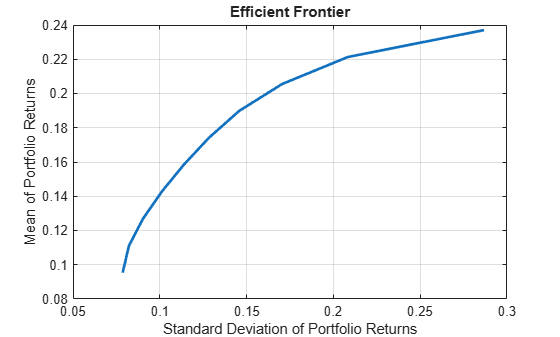# estimatePortMoments

Estimate moments of portfolio returns for Portfolio object

## Syntax

``````[prsk,pret] = estimatePortMoments(obj,pwgt)``````

## Description

example

``````[prsk,pret] = estimatePortMoments(obj,pwgt)``` estimate moments of portfolio returns for a `Portfolio` object. For details on the workflow, see Portfolio Object Workflow.The estimate of port moments is specific to mean-variance portfolio optimization and computes the mean and standard deviation (which is the square-root of variance) of portfolio returns.```

## Examples

collapse all

Given portfolio `p`, use the `estimatePortMoments` function to show the range of risks and returns for efficient portfolios.

```m = [ 0.05; 0.1; 0.12; 0.18 ]; C = [ 0.0064 0.00408 0.00192 0; 0.00408 0.0289 0.0204 0.0119; 0.00192 0.0204 0.0576 0.0336; 0 0.0119 0.0336 0.1225 ]; p = Portfolio; p = setAssetMoments(p, m, C); p = setDefaultConstraints(p); pwgt = estimateFrontierLimits(p); [prsk, pret] = estimatePortMoments(p, pwgt); disp([prsk, pret]);```
``` 0.0769 0.0590 0.3500 0.1800 ```

Create a `Portfolio` object for three assets.

```AssetMean = [ 0.0101110; 0.0043532; 0.0137058 ]; AssetCovar = [ 0.00324625 0.00022983 0.00420395; 0.00022983 0.00049937 0.00019247; 0.00420395 0.00019247 0.00764097 ]; p = Portfolio('AssetMean', AssetMean, 'AssetCovar', AssetCovar); p = setDefaultConstraints(p); ```

Use `setBounds` with semi-continuous constraints to set xi=`0` or `0.02`<=`xi`<=`0.5` for all i=`1`,...`NumAssets.`

`p = setBounds(p, 0.02, 0.5,'BoundType', 'Conditional', 'NumAssets', 3); `

When working with a `Portfolio` object, the `setMinMaxNumAssets` function enables you to set up cardinality constraints for a long-only portfolio. This sets the cardinality constraints for the `Portfolio` object, where the total number of allocated assets satisfying the nonzero semi-continuous constraints are between `MinNumAssets` and `MaxNumAssets`. By setting `MinNumAssets`=`MaxNumAssets`=2, only two of the three assets are invested in the portfolio.

`p = setMinMaxNumAssets(p, 2, 2); `

Use `estimatePortMoments` to estimate moments of portfolio returns for a `Portfolio` object.

```pwgt = estimateFrontierLimits(p); [prsk, pret] = estimatePortMoments(p, pwgt)```
```prsk = 2×1 0.0324 0.0695 ```
```pret = 2×1 0.0072 0.0119 ```

The `estimatePortMoments` function uses the MINLP solver to solve this problem. Use the `setSolverMINLP` function to configure the `SolverType` and options.

`p.solverOptionsMINLP`
```ans = struct with fields: MaxIterations: 1000 AbsoluteGapTolerance: 1.0000e-07 RelativeGapTolerance: 1.0000e-05 NonlinearScalingFactor: 1000 ObjectiveScalingFactor: 1000 Display: 'off' CutGeneration: 'basic' MaxIterationsInactiveCut: 30 ActiveCutTolerance: 1.0000e-07 IntMasterSolverOptions: [1x1 optim.options.Intlinprog] NumIterationsEarlyIntegerConvergence: 30 ```

The `Portfolio` object is able to find an efficient portfolio with respect to a specified target risk.

Load a vector of expected returns and a covariance matrix for 30 stocks.

`load StockStats`

Create `Portfolio` Object with Default Constraints

The default constraints for a `Portfolio` object are that it is a long-only portfolio and that it is 100% invested. Many other constraint types are possible.

```P = Portfolio('mean',expRet,'covar',expCov); P = setDefaultConstraints(P);```

Plot Full Efficient Frontier

Use the `plotFrontier` function with the `Portfolio` object to visualize the full frontier. Other functions exist that allow you to probe into particular portfolios along the frontier.

`P.plotFrontier`Capture Upper and Lower Bounds of Portfolio Risks and Returns

It is useful to know what are the upper and lower limits of the portfolio moments along the efficient frontier. This information allows you to determine what are feasible targets. Use the `estimateFrontierLimits` function with the `Portfolio` object to identify the weights at these extremes. Then you can use the `estimatePortMoments` function `Portfolio` object to find the limiting moments.

```P_Weights1 = P.estimateFrontierLimits; [P_risklimits, P_returnlimits] = P.estimatePortMoments(P_Weights1)```
```P_risklimits = 2×1 0.0786 0.2868 ```
```P_returnlimits = 2×1 0.0954 0.2370 ```

Estimate Efficient Portfolio with Target Return

Select a target return for the portfolio somewhere in the feasible region. You can estimate its makeup with `estimateFrontierByRisk` and then confirm its moments using `estimatePortMoments`.

```targetReturn = 0.15; P_Weights2 = P.estimateFrontierByReturn(targetReturn); [P_risk2, P_return2] = P.estimatePortMoments(P_Weights2)```
```P_risk2 = 0.1068 ```
```P_return2 = 0.1500 ```

The return matches the `targetReturn` value and the risk is in agreement with the efficient frontier plot.

Estimate Efficient Portfolio with Target Risk

The `Portfolio` object is able to find an efficient portfolio with a specified target risk.

```targetRisk = 0.2; P_Weights3 = P.estimateFrontierByRisk(targetRisk); [P_risk3, P_return3] = P.estimatePortMoments(P_Weights3)```
```P_risk3 = 0.2000 ```
```P_return3 = 0.2182 ```

Another useful feature of the `estimateFrontierByReturn` and `estimateFrontierByRisk` functions is what happens if you specify an infeasible (too high or too low) target. In this case, these functions provide a warning message to suggest the best solution.

## Input Arguments

collapse all

Object for portfolio, specified using a `Portfolio` object. For more information on creating a portfolio object, see

Data Types: `object`

Collection of portfolios, specified as a `NumAssets`-by-`NumPorts` matrix where `NumAssets` is the number of assets in the universe and `NumPorts` is the number of portfolios in the collection of portfolios.

Data Types: `double`

## Output Arguments

collapse all

Estimates for standard deviations of portfolio returns for each portfolio in `pwgt`, returned as a `NumPorts` vector.

`prsk` is returned for a `Portfolio` input object (`obj`).

Estimates for means of portfolio returns for each portfolio in `pwgt`, returned as a `NumPorts` vector.

`pret` is returned for a `Portfolio` input object (`obj`).

## Tips

You can also use dot notation to estimate the moments of portfolio returns.

`[prsk, pret] = obj.estimatePortMoments(pwgt);`

## Version History

Introduced in R2011a